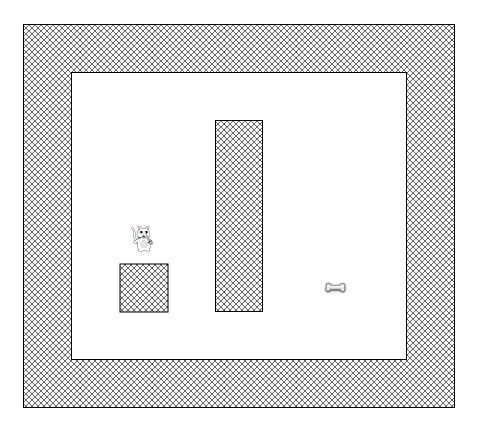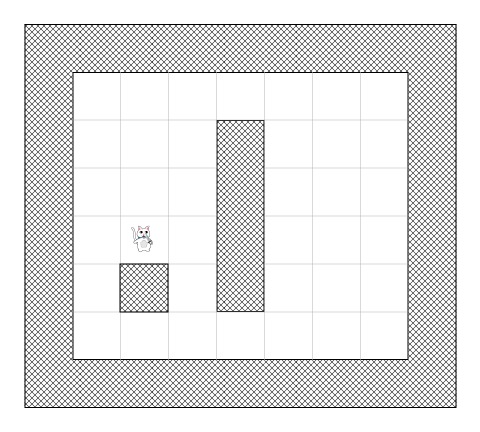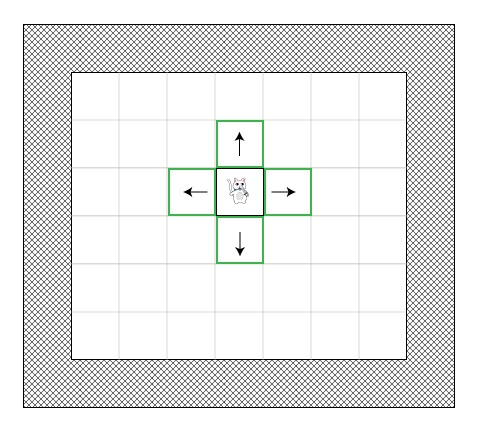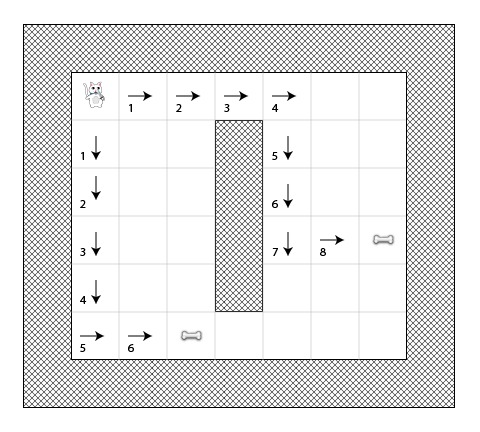# Introduction to A* Pathfinding

This is a blog post by iOS Tutorial Team member Johann Fradj, a software developer currently full-time dedicated to iOS. He is the co-founder of Hot Apps Factory which is the creator of App Cooker. Have you ever had a game where you wanted to make monsters or players move to a particular point, while […] By Ray Wenderlich.

Leave a rating/review
Save for later
Share

## Introduction to A* Pathfinding

20 mins

This is a blog post by iOS Tutorial Team member Johann Fradj, a software developer currently full-time dedicated to iOS. He is the co-founder of Hot Apps Factory which is the creator of App Cooker.

Have you ever had a game where you wanted to make monsters or players move to a particular point, while avoiding walls and obstacles?

If so, read this tutorial, and we’ll show you how you can do this with A* pathfinding!

There are already several articles on A* pathfinding on the web, but most of them are for experienced developers who already know the basics.

This tutorial takes the approach of covering the fundamentals from the ground up. We’ll discuss how the A* pathfinding algorithm works step-by-step, and include a ton of pictures and examples to diagram the process.

Regardless of what programming language or platform you are using, you should find this tutorial helpful as it explains the algorithm in a language agnostic format. Later on, we’ll have a follow-up tutorial that shows an example implementation in a Cocos2D iPhone game.

So find the shortest path to a caffeinated beverage and a tasty snack, and let’s begin! :]

## A Pathfinding Cat

Let’s imagine that we have a game where a cat wants to find a way to get a bone.

“Why in the world would a cat want a bone?!” you might think. Well in our game, this is a crafty cat and he wants to pick up bones to give to dogs, to avoid getting himself chomped! :]

So imagine the cat in the picture below wants to find the shortest path to the bone:Sadly, the cat can’t go straight from his current position to the bone, because there is a wall blocking the way, and he’s not a ghost cat in this game!

And the cat in this game is also quite lazy, so he always wants to find the shortest path so he’s not too tired when he gets back home to see his female ;-)

But how can we write an algorithm to figure out which path the cat should take? A* to the rescue!

## Simplifying the Search Area

The first step in pathfinding is to simplify the search area into something easily manageable.

How to do this depends on the game. For example, we could divide the search area into pixels, but that’s a granularity which is too high (and unnecessary) for our a tile-based game like this.

So instead, we will use the tile (a square shape) as unit for the pathfinding algorithm. Variants with other type of shapes are possible (such as triangles or hexagons), but the square is the best fit for our needs and is also the simplest.

Divided like that, our search area can be simply represented by a two dimensional array sized like the map it represents. So if the level is a map of 25*25 tiles, our search area will be an array of 625 squares. If we’ve divided our map into pixels, the search area would have to be an array of 640,000 squares (A tile is 32*32 pixels)!

So let’s take the screenshot we started with and divide it into tiles to represent the search area (in this simple example, 7×6 tiles = 42 tiles total):## The Open and Closed Lists

Now that we’ve created a simplified search area, let’s discuss how the A* algorithm works.

In addition to being lazy, our cat does not have a good memory, so it will need two lists:

1. One to write down all the squares that are being considered to find the shortest path (called the open list)
2. One to write down the square that does not have to consider it again (called the closed list

The cat begins by adding his current position (we’ll call this starting position point “A”) to the closed list. Then, he adds all walkable tiles adjacent to his current position to the open list.

Here’s an example of what this would look like if the cat was out in the open (green representing the open list):Now the cat need to determine which of these options would be on the shortest path, but how can he choose?

Well in the A* path algorithm, this is done by giving a score to each square, which is called path scoring. Let’s see how it works!

## Path Scoring

We’ll give each square a score G + H where:

• G is the movement cost from the start point A to the current square. So for a square adjacent to the start point A, this would be 1, but this will increase as we get farther away from the start point.
• H is the estimated movement cost from the current square to the destination point (we’ll call this point B for Bone!) This is often called the heuristic because we don’t really know the cost yet – it’s just an estimate.

You may be wondering what we mean by “movement cost”. Well in this game it will be quite simple – just the number of squares.

However, keep in mind that you can make this different for our game. For example:

• If you allowed diagonal movement, you might make the movement cost a bit bigger for diagonal moves.
• If you had different terrain types, you might make some cost more to move through – for example a swamp, water, or a Catwoman poster ;-)

That’s the general idea – now let’s dive into more specifics about figuring out G and H.

Recall that G is the movement cost (in number of squares for this game) from the start point A to the current square.

In order to calculate G, we need to take the G of its parent (the square where we came from) and to add 1 to it. Therefore, the G of each square will represent the total cost of the generated path from point A until the square.

For example, this diagram shows two paths to two different bones, with the G score of each square listed on the square:Ray Wenderlich

Author